# sklearn.inspection.permutation_importance¶

sklearn.inspection.permutation_importance(estimator, X, y, *, scoring=None, n_repeats=5, n_jobs=None, random_state=None, sample_weight=None, max_samples=1.0)[source]

Permutation importance for feature evaluation [BRE].

The estimator is required to be a fitted estimator. X can be the data set used to train the estimator or a hold-out set. The permutation importance of a feature is calculated as follows. First, a baseline metric, defined by scoring, is evaluated on a (potentially different) dataset defined by the X. Next, a feature column from the validation set is permuted and the metric is evaluated again. The permutation importance is defined to be the difference between the baseline metric and metric from permutating the feature column.

Read more in the User Guide.

Parameters:
estimatorobject

An estimator that has already been fitted and is compatible with scorer.

Xndarray or DataFrame, shape (n_samples, n_features)

Data on which permutation importance will be computed.

yarray-like or None, shape (n_samples, ) or (n_samples, n_classes)

Targets for supervised or None for unsupervised.

scoringstr, callable, list, tuple, or dict, default=None

Scorer to use. If scoring represents a single score, one can use:

If scoring represents multiple scores, one can use:

• a list or tuple of unique strings;

• a callable returning a dictionary where the keys are the metric names and the values are the metric scores;

• a dictionary with metric names as keys and callables a values.

Passing multiple scores to scoring is more efficient than calling permutation_importance for each of the scores as it reuses predictions to avoid redundant computation.

If None, the estimator’s default scorer is used.

n_repeatsint, default=5

Number of times to permute a feature.

n_jobsint or None, default=None

Number of jobs to run in parallel. The computation is done by computing permutation score for each columns and parallelized over the columns. None means 1 unless in a joblib.parallel_backend context. -1 means using all processors. See Glossary for more details.

random_stateint, RandomState instance, default=None

Pseudo-random number generator to control the permutations of each feature. Pass an int to get reproducible results across function calls. See Glossary.

sample_weightarray-like of shape (n_samples,), default=None

Sample weights used in scoring.

New in version 0.24.

max_samplesint or float, default=1.0

The number of samples to draw from X to compute feature importance in each repeat (without replacement).

• If int, then draw max_samples samples.

• If float, then draw max_samples * X.shape samples.

• If max_samples is equal to 1.0 or X.shape, all samples will be used.

While using this option may provide less accurate importance estimates, it keeps the method tractable when evaluating feature importance on large datasets. In combination with n_repeats, this allows to control the computational speed vs statistical accuracy trade-off of this method.

New in version 1.0.

Returns:
resultBunch or dict of such instances

Dictionary-like object, with the following attributes.

importances_meanndarray of shape (n_features, )

Mean of feature importance over n_repeats.

importances_stdndarray of shape (n_features, )

Standard deviation over n_repeats.

importancesndarray of shape (n_features, n_repeats)

Raw permutation importance scores.

If there are multiple scoring metrics in the scoring parameter result is a dict with scorer names as keys (e.g. ‘roc_auc’) and Bunch objects like above as values.

References

Examples

>>> from sklearn.linear_model import LogisticRegression
>>> from sklearn.inspection import permutation_importance
>>> X = [[1, 9, 9],[1, 9, 9],[1, 9, 9],
...      [0, 9, 9],[0, 9, 9],[0, 9, 9]]
>>> y = [1, 1, 1, 0, 0, 0]
>>> clf = LogisticRegression().fit(X, y)
>>> result = permutation_importance(clf, X, y, n_repeats=10,
...                                 random_state=0)
>>> result.importances_mean
array([0.4666..., 0.       , 0.       ])
>>> result.importances_std
array([0.2211..., 0.       , 0.       ])


## Examples using sklearn.inspection.permutation_importance¶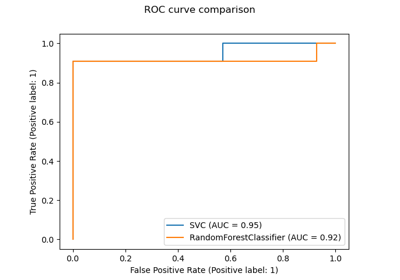Release Highlights for scikit-learn 0.22

Release Highlights for scikit-learn 0.22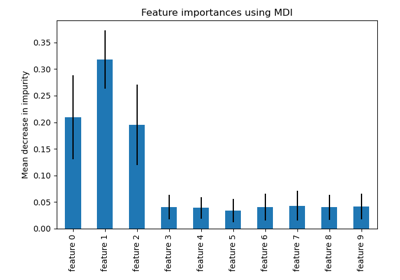Feature importances with a forest of trees

Feature importances with a forest of trees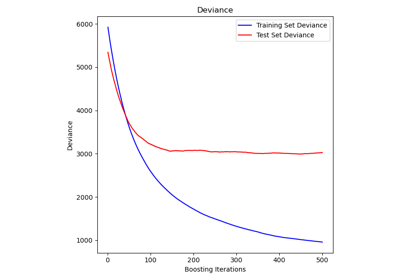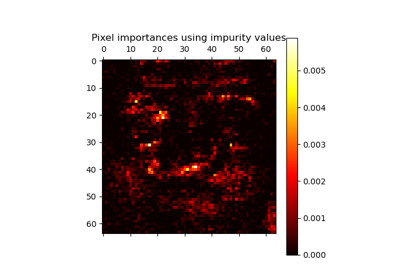Pixel importances with a parallel forest of trees

Pixel importances with a parallel forest of trees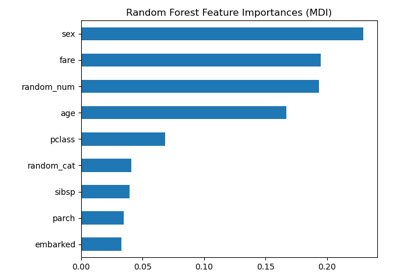Permutation Importance vs Random Forest Feature Importance (MDI)

Permutation Importance vs Random Forest Feature Importance (MDI)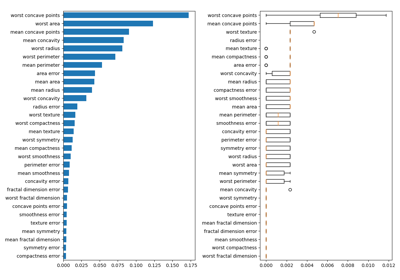Permutation Importance with Multicollinear or Correlated Features

Permutation Importance with Multicollinear or Correlated Features